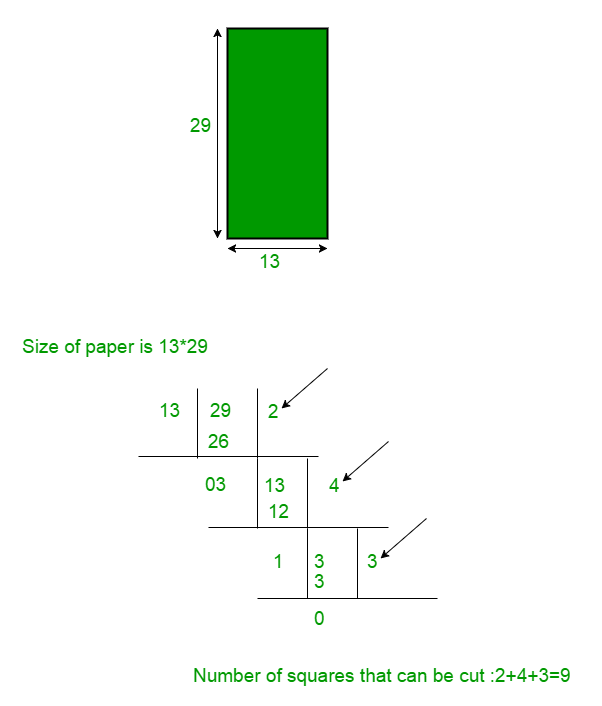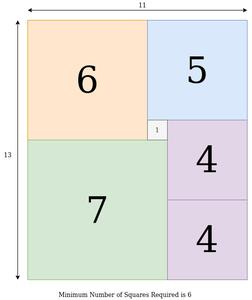# Paper Cut into Minimum Number of Squares | Set 2

Given a paper of size A x B. Task is to cut the paper into squares of any size. Find the minimum number of squares that can be cut from the paper.

Examples:

```Input  : 36 x 30
Output : 5
Explanation :
3 (squares of size 12x12) +
2 (squares of size 18x18)

Input  : 4 x 5
Output : 5
Explanation :
1 (squares of size 4x4) +
4 (squares of size 1x1)```

Recommended Practice

We have already discussed the Greedy approach to solve this problem in previous article. But the previous approach doesn’t always work. For example, it fails for the above first test case. So, in this article we solve this problem using Dynamic Programming.

We know that if we want to cut minimum number of squares from the paper then we would have to cut largest square possible from the paper first and largest square will have same side as smaller side of the paper. For example if paper have the size 13 x 29, then maximum square will be of side 13. so we can cut 2 square of size 13 x 13 (29/13 = 2). Now remaining paper will have size 3 x 13. Similarly we can cut remaining paper by using 4 squares of size 3 x 3 and 3 squares of 1 x 1. So minimum 9 squares can be cut from the Paper of size 13 x 29.Explanation
minimumSquare is a function which tries to split the rectangle at some position. The function is called recursively for both parts. Try all possible splits and take the one with minimum result. The base case is when both sides are equal i.e the input is already a square, in which case the result is We are trying to find the cut which is nearest to the center which will lead us to our minimum result.

Assuming we have a rectangle with width is N and height is M.

• if (N == M), so it is a square and nothing need to be done.
• Otherwise, we can divide the rectangle into two other smaller one (N – x, M) and (x, M), so it can be solved recursively.
• Similarly, we can also divide it into (N, M – x) and (N, x)

Also we need to be aware of an edge case here which is N=11 and M=13 or vice versa. The following will be the best possible answer for the given test case:Our Approach will return 8 for this case but as you can see we can cut the paper in 6 squares which is minimum. This happens because there is no vertical or horizontal line that cuts through the whole square in the optimum solution.

Below is the implementation of the above idea using Dynamic Programming.

## C++

 `// C++ program to find minimum number of squares` `// to cut a paper using Dynamic Programming` `#include` `using` `namespace` `std;`   `const` `int` `MAX = 300;` `int` `dp[MAX][MAX];`   `// Returns min number of squares needed` `int` `minimumSquare(``int` `m, ``int` `n)` `{` `    ``// Initializing max values to vertical_min ` `    ``// and horizontal_min` `    ``int` `vertical_min = INT_MAX;` `    ``int` `horizontal_min = INT_MAX;` `  `  `    ``// N=11 & M=13 is a special case` `    ``if``(n==13 && m==11) ``return` `6;` `    ``if``(m==13 && n==11) ``return` `6;` `    `  `    ``// If the given rectangle is already a square` `    ``if` `(m == n)` `        ``return` `1;` `    `  `    ``// If the answer for the given rectangle is ` `    ``// previously calculated return that answer` `    ``if` `(dp[m][n])` `            ``return` `dp[m][n];` `    `  `    ``/* The rectangle is cut horizontally and ` `       ``vertically into two parts and the cut ` `       ``with minimum value is found for every ` `       ``recursive call. ` `    ``*/` `    `  `    ``for` `(``int` `i = 1;i<= m/2;i++)` `    ``{` `        ``// Calculating the minimum answer for the ` `        ``// rectangles with width equal to n and length ` `        ``// less than m for finding the cut point for ` `        ``// the minimum answer` `        ``horizontal_min = min(minimumSquare(i, n) + ` `                ``minimumSquare(m-i, n), horizontal_min); ` `    ``}` `    `  `    ``for` `(``int` `j = 1;j<= n/2;j++)` `    ``{` `        ``// Calculating the minimum answer for the ` `        ``// rectangles with width less than n and ` `        ``// length equal to m for finding the cut ` `        ``// point for the minimum answer` `        ``vertical_min = min(minimumSquare(m, j) + ` `                ``minimumSquare(m, n-j), vertical_min);` `    ``}` `        `  `    ``// Minimum of the vertical cut or horizontal ` `    ``// cut to form a square is the answer` `    ``dp[m][n] = min(vertical_min, horizontal_min); ` `        `  `    ``return` `dp[m][n];` `}`   `// Driver code` `int` `main()` `{` `    ``int` `m = 30, n = 35;` `  `  `    ``// Function call` `    ``cout << minimumSquare(m, n);` `    ``return` `0;` `}`   `// This code is contributed by Utkarsh Gupta`

## Java

 `// Java program to find minimum number of` `// squares to cut a paper using Dynamic` `// Programming` `import` `java.io.*;`   `class` `GFG {`   `    ``static` `int` `dp[][] = ``new` `int``[``300``][``300``];`   `    ``// Returns min number of squares needed` `    ``static` `int` `minimumSquare(``int` `m, ``int` `n)` `    ``{` `        ``// Initializing max values to` `        ``// vertical_min and horizontal_min` `        ``int` `vertical_min = Integer.MAX_VALUE;` `        ``int` `horizontal_min = Integer.MAX_VALUE;` `      `  `        ``// N=11 & M=13 is a special case` `        ``if``(n==``13` `&& m==``11``) ``return` `6``;` `        ``if``(m==``13` `&& n==``11``) ``return` `6``;`   `        ``// If the given rectangle is` `        ``// already a square` `        ``if` `(m == n)` `            ``return` `1``;`   `        ``// If the answer for the given` `        ``// rectangle is previously` `        ``// calculated return that answer` `        ``if` `(dp[m][n] != ``0``)` `            ``return` `dp[m][n];`   `        ``/* The rectangle is cut horizontally` `        ``and vertically into two parts and` `        ``the cut with minimum value is found` `        ``for every recursive call.` `        ``*/`   `        ``for` `(``int` `i = ``1``; i <= m / ``2``; i++)` `        ``{` `            ``// Calculating the minimum answer` `            ``// for the rectangles with width` `            ``// equal to n and length less than` `            ``// m for finding the cut point for` `            ``// the minimum answer` `            ``horizontal_min` `                ``= Math.min(minimumSquare(i, n)` `                           ``+ minimumSquare(m - i, n),` `                           ``horizontal_min);` `        ``}`   `        ``for` `(``int` `j = ``1``; j <= n / ``2``; j++) {` `            ``// Calculating the minimum answer` `            ``// for the rectangles with width` `            ``// less than n and length equal to` `            ``// m for finding the cut point for` `            ``// the minimum answer` `            ``vertical_min` `                ``= Math.min(minimumSquare(m, j)` `                           ``+ minimumSquare(m, n - j),` `                           ``vertical_min);` `        ``}`   `        ``// Minimum of the vertical cut or` `        ``// horizontal cut to form a square` `        ``// is the answer` `        ``dp[m][n] = Math.min(vertical_min, horizontal_min);`   `        ``return` `dp[m][n];` `    ``}`   `    ``// Driver code` `    ``public` `static` `void` `main(String[] args)` `    ``{` `        ``int` `m = ``30``, n = ``35``;` `      `  `        ``// Function call` `        ``System.out.println(minimumSquare(m, n));` `    ``}` `}` `// This code is contributed by Prerna Saini`

## Python3

 `# Python3 program to find minimum` `# number of squares` `# to cut a paper using Dynamic Programming`   `MAX` `=` `300` `dp ``=` `[[``0` `for` `i ``in` `range``(``MAX``)] ``for` `i ``in` `range``(``MAX``)]`   `# Returns min number of squares needed`     `def` `minimumSquare(m, n):`   `    ``# Initializing max values to` `    ``# vertical_min` `    ``# and horizontal_min` `    ``vertical_min ``=` `10000000000` `    ``horizontal_min ``=` `10000000000`   `    ``# N=11 & M=13 is a special case` `    ``if` `n ``=``=` `13` `and` `m ``=``=` `11``:` `        ``return` `6` `    ``if` `m ``=``=` `13` `and` `n ``=``=` `11``:` `        ``return` `6`   `    ``# If the given rectangle is` `    ``# already a square` `    ``if` `m ``=``=` `n:` `        ``return` `1`   `    ``# If the answer for the given rectangle is` `    ``# previously calculated return that answer` `    ``if` `dp[m][n] !``=` `0``:` `        ``return` `dp[m][n]`   `    ``# The rectangle is cut horizontally and` `    ``# vertically into two parts and the cut` `    ``# with minimum value is found for every` `    ``# recursive call.` `    ``for` `i ``in` `range``(``1``, m``/``/``2``+``1``):`   `        ``# Calculating the minimum answer for the` `        ``# rectangles with width equal to n and length` `        ``# less than m for finding the cut point for` `        ``# the minimum answer` `        ``horizontal_min ``=` `min``(minimumSquare(i, n) ``+` `                             ``minimumSquare(m``-``i, n), horizontal_min)` `    ``for` `j ``in` `range``(``1``, n``/``/``2``+``1``):`   `        ``# Calculating the minimum answer for the` `        ``# rectangles with width equal to n and length` `        ``# less than m for finding the cut point for` `        ``# the minimum answer` `        ``vertical_min ``=` `min``(minimumSquare(m, j) ``+` `                           ``minimumSquare(m, n``-``j), vertical_min)`   `    ``# Minimum of the vertical cut or horizontal` `    ``# cut to form a square is the answer` `    ``dp[m][n] ``=` `min``(vertical_min, horizontal_min)` `    ``return` `dp[m][n]`     `# Driver code` `if` `__name__ ``=``=` `'__main__'``:` `    ``m ``=` `30` `    ``n ``=` `35`   `    ``# Function call` `    ``print``(minimumSquare(m, n))`   `# This code is contributed by sahilshelangia`

## C#

 `// C# program to find minimum number of` `// squares to cut a paper using Dynamic` `// Programming` `using` `System;`   `class` `GFG {`   `    ``static` `int``[, ] dp = ``new` `int``[300, 300];`   `    ``// Returns min number of squares needed` `    ``static` `int` `minimumSquare(``int` `m, ``int` `n)` `    ``{` `        ``// Initializing max values to` `        ``// vertical_min and horizontal_min` `        ``int` `vertical_min = ``int``.MaxValue;` `        ``int` `horizontal_min = ``int``.MaxValue;` `      `  `        ``// N=11 & M=13 is a special case` `        ``if``(n==13 && m==11) ``return` `6;` `        ``if``(m==13 && n==11) ``return` `6;`   `        ``// If the given rectangle is` `        ``// already a square` `        ``if` `(m == n)` `            ``return` `1;`   `        ``// If the answer for the given` `        ``// rectangle is previously` `        ``// calculated return that answer` `        ``if` `(dp[m, n] != 0)` `            ``return` `dp[m, n];`   `        ``/* The rectangle is cut horizontally` `        ``and vertically into two parts and` `        ``the cut with minimum value is found` `        ``for every recursive call.` `        ``*/`   `        ``for` `(``int` `i = 1; i <= m / 2; i++) ` `        ``{` `            ``// Calculating the minimum answer` `            ``// for the rectangles with width` `            ``// equal to n and length less than` `            ``// m for finding the cut point for` `            ``// the minimum answer` `            ``horizontal_min` `                ``= Math.Min(minimumSquare(i, n)` `                               ``+ minimumSquare(m - i, n),` `                           ``horizontal_min);` `        ``}`   `        ``for` `(``int` `j = 1; j <= n / 2; j++) ` `        ``{` `            ``// Calculating the minimum answer` `            ``// for the rectangles with width` `            ``// less than n and length equal to` `            ``// m for finding the cut point for` `            ``// the minimum answer` `            ``vertical_min` `                ``= Math.Min(minimumSquare(m, j)` `                               ``+ minimumSquare(m, n - j),` `                           ``vertical_min);` `        ``}`   `        ``// Minimum of the vertical cut or` `        ``// horizontal cut to form a square` `        ``// is the answer` `        ``dp[m, n] = Math.Min(vertical_min, horizontal_min);`   `        ``return` `dp[m, n];` `    ``}`   `    ``// Driver code` `    ``public` `static` `void` `Main()` `    ``{` `        ``int` `m = 30, n = 35;`   `        ``// Function call` `        ``Console.WriteLine(minimumSquare(m, n));` `    ``}` `}`   `// This code is contributed by anuj_67.`

## Javascript

 ``

Output

`5`

Time Complexity: O(N * M * (N + M))

Space Complexity: O(N * M)

If you like GeeksforGeeks and would like to contribute, you can also write an article using write.geeksforgeeks.org or mail your article to review-team@geeksforgeeks.org. See your article appearing on the GeeksforGeeks main page and help other Geeks.